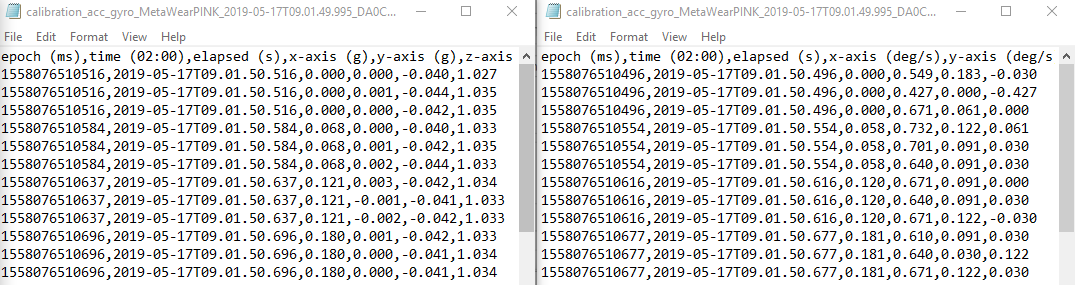# Logging accelerations and angular velocity (MetaTracker) - How to read the "elapsed" data column

Dear all,

I have run a simple test with a single MetaTracker, when it was lying still on my desk. I have established a connection with the device using MetaBase for Windows 10.
I have set the sensor settings to log accelerations (50 Hz, +/- 8g) and angular velocity (50 Hz, +/- 1000 deg/s) (see: attachment).

When I look at the data, I get the following (accelerometer on the left, gyroscope on the right):Now, I was wondering how I should read the `elapsed (s)` data column.

A sampling frequency of 50 Hz would mean that a sample is captured every 0.02 seconds. Thus, I would expect an `elapsed (s)` column to be [0.000, 0.020, 0.040, ...]T

However, as can be seen from the log I have 3 samples at time 0.000, and then 3 samples at 0.068, then 3 samples at 0.121 (at least, for the accelerometer). This means that every third sample is more or less captured at the expected time, can I just interpolate the elapsed times for the two samples in between?

Also, the gyroscope output is not synchronized completely with the accelerometer. Can I just assume here that they are more or less from the same timestamp? For example, samples 4, 5, 6 are captured at time 0.068 seconds (accelerations) or 0.058 seconds (angular velocities). This means accelerations and angular velocities are 0.01 seconds out-of-phase for a sampling period of 0.02 second.

Kind regards,
Robbin